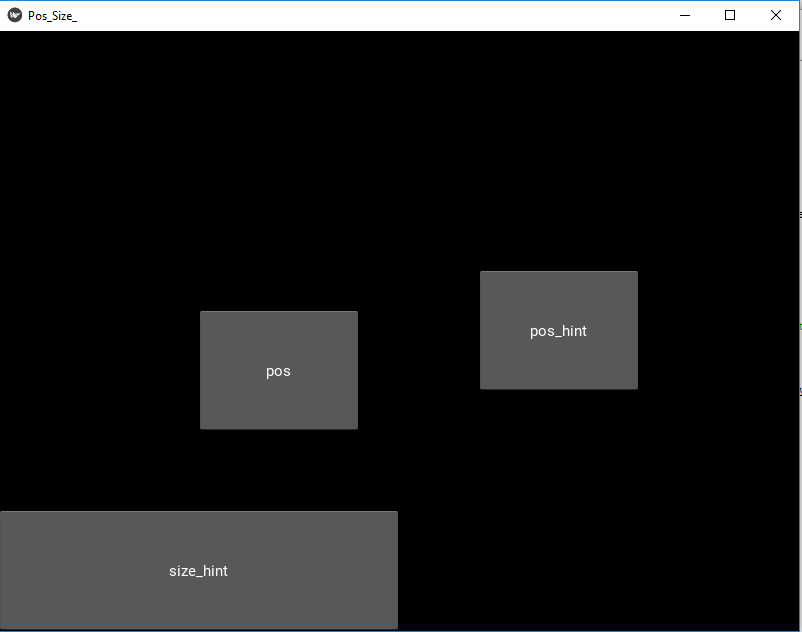# Change the size and position of button in Kivy

• Last Updated : 19 Oct, 2021

Kivy is a platform independent GUI tool in Python. As it can be run on Android, IOS, linux and Windows etc. It is basically used to develop the Android application, but it does not mean that it can not be used on Desktops applications.
In this article, we will see that how can we can change the size and the position of button in kivy Python. There are 4 properties to set up, the size and position of button. There are 2 properties which are for static placement and another 2 are for dynamic placement.

size : It takes two arguments i.e. (width, height).

## Python3

 `b1 ``=` `Button(size ``=``(``100``, ``100``))``b2 ``=` `Button(size ``=``(``200``, ``200``))`

pos : pos stands for position i.e it is used to position the widget. By default (0, 0), the bottom-left corner of the screen is the default position of the button in kivy python.

## Python3

 `b1 ``=` `Button(pos ``=``(``100``, ``100``))``b2 ``=` `Button(pos ``=``(``200``, ``200``))`

size_hint : Provide hint of size. It contains two arguments i.e. width and height it can be floating values. By default, all widgets have their size_hint=(1, 1). To create a button 50% of the width and 25% of the height of the layout and positioned at (20, 20), you can do –

## Python3

 `button ``=` `Button(``    ``text ``=``'Hello world'``,``    ``size_hint ``=``(.``5``, .``25``),``    ``pos ``=``(``20``, ``20``))`

pos_hint : Provide hint of position. We can define upto 8 keys i.e. it takes arguments in form of dictionary.

```pos_hint = {“x”:1, “y”:1, “left”:1, “right”:1, "center_x":1,
"center_y":1, "top”:1, “bottom”:1("top":0)}```

If you want to create a button that will always be the size of layout minus 20% on each side –

## Python3

 `button ``=` `Button(text ``=``'Hello world'``, size_hint ``=``(.``6``, .``6``),``                ``pos_hint ``=``{``'x'``:.``2``, ``'y'``:.``2``})`

Kivy Tutorial – Learn Kivy with Examples.

Note:

• You can only use values between 0-1 for both size_hint and pos_hint. Where 0 = 0% and 1 = 100%.
• The coordinate system in kivy works from the bottom left! This will be important when placing our objects. (i.e (0, 0) is the bottom left).

```Basic Approach:

1) import kivy
2) import kivyApp
3) import all neaded(like button and layouts to use them)
4) Set minimum version(optional)
5) create Layout class
6) create App class
7) Set up the position and size of the buttons
8) return Layout/widget/Class(according to requirement)
9) Run an instance of the class```

Below is the implementation of all 4 properties:

## Python3

 `## Sample Python application demonstrating the``## How to change button position and size in Kivy.``###################################################``# import modules``import` `kivy` `# base Class of your App inherits from the App class.``# app:always refers to the instance of your application``from` `kivy.app ``import` `App` `# creates the button in kivy``# if not imported shows the error``from` `kivy.uix.button ``import` `Button` `# This layout allows you to set relative coordinates for children.``from` `kivy.uix.relativelayout ``import` `RelativeLayout` `# To change the kivy default settings``# we use this module config``from` `kivy.config ``import` `Config``    ` `# 0 being off 1 being on as in true / false``# you can use 0 or 1 && True or False``Config.``set``(``'graphics'``, ``'resizable'``, ``True``)` `# creating the App class``class` `Pos_Size_App(App):``    ` `    ``def` `build(``self``):` `        ``# A Relative Layout with a size of (300, 300) is created``        ``rl ``=` `RelativeLayout(size ``=``(``300``, ``300``))``        ` `        ``# creating button``        ``# size of button is 20 % by height and width of layout``        ``# position is 'center_x':.7, 'center_y':.5``        ``b1 ``=` `Button(size_hint ``=``(.``2``, .``2``),``                    ``pos_hint ``=``{``'center_x'``:.``7``, ``'center_y'``:.``5``},``                    ``text ``=``"pos_hint"``)` `        ``# creating button``        ``# size of button is 20 % by height and 50 % width of layout``        ``b2 ``=` `Button(size_hint ``=``(.``5``, .``2``), ``                    ``text ``=``"size_hint"``)` `                ``# creating button``        ``# size of button is 20 % by height and width of layout``        ``# position is 200, 200 from bottom left``        ``b3 ``=` `Button( size_hint ``=``(.``2``, .``2``),``                    ``pos ``=``(``200``, ``200``),``                    ``text ``=``"pos"``)``        ` `        `  `        ``# adding button to widget``        ``rl.add_widget(b1)``        ``rl.add_widget(b2)``        ``rl.add_widget(b3)``    ` `        ` `        ``# returning widget``        ``return` `rl` `# run the App``if` `__name__ ``=``=` `"__main__"``:``    ``Pos_Size_App().run()`

Output:My Personal Notes arrow_drop_up